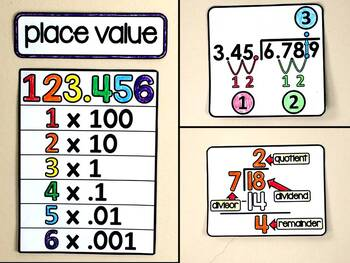# 5th Grade Math Word Wall - print and digital5th
Subjects
Standards
Resource Type
Formats Included
• PDF
•Google Apps™
Pages
75 pagesThe Teacher-Author indicated this resource includes assets from Google Workspace (e.g. docs, slides, etc.).

#### Also included in

1. This bundle contains every math word wall-- grades 2 through 8, algebra, geometry, algebra 2, the unit circle, area, volume, integers and financial literacy-- so that you can customize your math vocabulary display based on the needs of your students throughout the year and year to year.Every word wa
\$80.00
\$149.00
Save \$69.00
2. This word wall bundle includes print and digital math word walls covering standards from 5th grade, 6th grade, 7th grade and 8th grade. All of the topics covered are listed in the preview file and standards can be seen in each individual word wall listing. The word walls in this bundle show math voc
\$37.50
\$53.00
Save \$15.50
3. This word wall bundle includes print and digital math word walls covering standards from 4th grade, 5th grade, 6th grade and 7th grade. All of the topics covered are listed in the preview file and standards can be seen in each individual word wall listing. The word walls in this bundle show math voc
\$37.50
\$53.00
Save \$15.50
4. This word wall bundle includes print and digital math word walls covering standards from 3rd grade, 4th grade, 5th grade and 6th grade. All of the topics covered are listed in the preview file and standards can be seen in each individual word wall listing. The word walls in this bundle show math voc
\$37.50
\$53.00
Save \$15.50
5. This word wall bundle includes print and digital math word walls covering standards from 2nd grade, 3rd grade, 4th grade and 5th grade. All of the topics covered are listed in the preview file and standards can be seen in each individual word wall listing. The word walls in this bundle show math voc
\$37.50
\$53.00
Save \$15.50

### Description

With references for rounding, place value, additive pattern, multiplicative pattern, coordinates, powers of 10, metric measurements, adding, subtracting, multiplying and dividing decimals, decimals vs. their fractions, adding fractions, subtracting fractions, multiplying fractions by whole numbers, dividing fractions by whole numbers, dividend, divisor, quotient, remainder, PEMDAS, numeric and algebraic expressions, improper fractions, proper fractions, mixed numbers, simplifying, and classifying quadrilaterals, this word wall is a great addition to a 5th grade math classroom.

*Spanish vocabulary words now included.

For the printable word wall, everything prints on 8.5x11 computer paper. You can choose to print in color or in black and white. Both are included. In the file are closeup photos of the word wall to use as an assembly guide.

The no-prep digital word wall is in Google Slides and is fully interactive as part of your virtual classroom. Each image on the home wall clicks to an enlarged image where there is also a whiteboard. On the whiteboard you can type notes, add video links, add more references, etc., based on what you feel is best for your students. Or you can send the file to students as-is. Students can refer to their digital word wall while working online or you can use the digital version as a teaching tool in your classroom.

You may also like:

6th Grade Math Word Wall - print and digital

Place Value Math Pennant

Decimal Review Digital Math Escape Room

Total Pages
75 pages
N/A
Teaching Duration
1 Year
Report this Resource to TpT
Reported resources will be reviewed by our team. Report this resource to let us know if this resource violates TpT’s content guidelines.

### Standards

to see state-specific standards (only available in the US).
Use parentheses, brackets, or braces in numerical expressions, and evaluate expressions with these symbols.
Generate two numerical patterns using two given rules. Identify apparent relationships between corresponding terms. Form ordered pairs consisting of corresponding terms from the two patterns, and graph the ordered pairs on a coordinate plane. For example, given the rule “Add 3” and the starting number 0, and given the rule “Add 6” and the starting number 0, generate terms in the resulting sequences, and observe that the terms in one sequence are twice the corresponding terms in the other sequence. Explain informally why this is so.
Add and subtract fractions with unlike denominators (including mixed numbers) by replacing given fractions with equivalent fractions in such a way as to produce an equivalent sum or difference of fractions with like denominators. For example, 2/3 + 5/4 = 8/12 + 15/12 = 23/12. (In general, 𝘢/𝘣 + 𝘤/𝘥 = (𝘢𝘥 + 𝘣𝘤)/𝘣𝘥.)
Interpret a fraction as division of the numerator by the denominator (𝘢/𝘣 = 𝘢 ÷ 𝘣). Solve word problems involving division of whole numbers leading to answers in the form of fractions or mixed numbers, e.g., by using visual fraction models or equations to represent the problem. For example, interpret 3/4 as the result of dividing 3 by 4, noting that 3/4 multiplied by 4 equals 3, and that when 3 wholes are shared equally among 4 people each person has a share of size 3/4. If 9 people want to share a 50-pound sack of rice equally by weight, how many pounds of rice should each person get? Between what two whole numbers does your answer lie?
Apply and extend previous understandings of multiplication to multiply a fraction or whole number by a fraction.